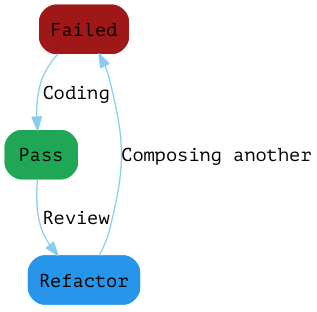示例的需求描述#

1. hmm经过处理之后，应该保持原状
2. she经过处理之后，应该被替换为shmommy
3. hear经过处理之后，应该被替换为hmommyr

TDD步骤#

1. 先编写一个测试，由于此时没有任何实现，因此测试会失败
2. 编写实现，以最快，最简单的方式，此时测试会通过
3. 查看实现/测试，有没有改进的余地，如果有的话就用重构的方式来优化，并在重构之后保证测试通过1. 时时关注于实现功能，这样不会跑偏
2. 每个功能都有测试覆盖，一旦改错，就会有测试失败
3. 重构时更有信心，不用怕破坏掉已有的功能
4. 测试即文档，而且是不会过期的文档，因为一旦实现变化，相关测试就会失败

1. 输入一个非元音字符，并预期返回字符本身
2. 输入一个元音，并预期返回mommy
3. 输入一个元音超过30%的字符串，并预期元音被替换
4. 输入一个元音超过30%，并且存在连续元音的字符串，并预期只被替换一次

实践#

第一个任务#

describe("mommify", function() {
it("should return h when given h", function() {
var expected = "h";

var result = mommify("h");

expect(result).toEqual(expected);
});
});

1. 组织数据（Arrange）
2. 执行需要被测的函数（Action）
3. 验证结果（Assertion）

function mommify() {
return "h";
}

it("should return m when given m", function() {
var expected = "m";

var result = mommify("m");

expect(result).toEqual(expected);
});

function mommify(word) {
return word;
}

第二个任务#

it("should return mommy when given a", function() {
var expected = "mommy";

var result = mommify("a");

expect(result).toEqual(expected);
});

function mommify(word) {
if(word == "a") {
return "mommy";
}
return word;
}

it("should return mommy when given a vowel", function() {
var expected = "mommy";

["a", "e", "i", "o", "u"].forEach(function(word) {
var result = mommify(word);
expect(result).toEqual(expected);
});
});

function mommify(word) {
if(word == "a" || word == "e" || word == "i" || word == "o" || word == "u") {
return "mommy";
}
return word;
}

function mommify(word) {
if("aeiou".indedOf(word) >= 0) {
return "mommy";
}
return word;
}

第三个任务#

it("should mommify if the vowels greater than 30%", function() {
var expected = "shmommy";
var result = mommify("she");

expect(result).toEqual(expected);
});

function mommify(word) {
var count = 0;
for(var i = 0; i < word.length; i++) {
if("aeiou".indexOf(word[i]) >= 0) {
count += 1;
}
}

var str = "";

if(count/word.length >= 0.30) {
for(var i = 0; i < word.length; i++) {
if("aeiou".indexOf(word[i]) >= 0) {
str += "mommy";
} else {
str += word[i];
}
}
} else {
str = word;
}

return str;
}

function countVowels(word) {
var count = 0;

for(var i = 0; i < word.length; i++) {
if("aeiou".indexOf(word[i]) >= 0) {
count += 1;
}
}

return count;
}

function isVowel(character) {
return "aeiou".indexOf(character) >= 0;
}

function mommify(word) {
var count = countVowels(word);
var str = "";

if(count/word.length >= 0.30) {
for(var i = 0; i < word.length; i++) {
if(isVowel(word[i])) {
str += "mommy";
} else {
str += word[i];
}
}
} else {
str = word;
}

return str;
}

function shouldBeMommify(word) {
var count = countVowels(word);
return count/word.length >= 0.30;
}

function replace(word) {
var str = "";

for(var i = 0; i < word.length; i++) {
if(isVowel(word[i])) {
str += "mommy";
} else {
str += word[i];
}
}

return str;
}

function mommify(word) {
if(shouldBeMommify(word)) {
return replace(word);
} else {
return word;
}
}

第四个任务#

it("should not mommify if there are vowels sequences", function() {
var expected = "shmommyr";
var result = mommify("shear");

expect(result).toEqual(expected);
});

function replace(word) {
var str = "";

for(var i = 0; i < word.length; i++) {
if(isVowel(word[i])) {
if(!isVowel(word[i-1])) {
str += "mommy";
} else {
str += "";
}
} else {
str += word[i];
}
}

return str;
}

function next(current, previous) {
var next = "";

if(isVowel(current)) {
if(!isVowel(previous)) {
next = "mommy";
}
} else {
next = current;
}

return next;
}

function replace(word) {
var str = "";

for(var i = 0; i < word.length; i++) {
str += next(word[i], word[i-1]);
}

return str;
}

结束（？）#

TDD是一种容易付诸实践的开发方式，在小的，简单的例子上如此，在大的，复杂的场景下也是如此。它优美且高效的地方在于：不假设任何人可以一次就写出完善的应用，而是鼓励小步前进，快速反馈，快速迭代。而演化到最后，得到的往往就是孜孜以求的优美设计。